# 20202309 葛鹏宇 《数据结构与面向对象程序设计》实验7实验报告

# 学号20202309  2021-2022-1 《数据结构与面向对象程序设计》实验2报告

## 1.实验内容

1. 定义一个Searching和Sorting类，并在类中实现linearSearch,SelectionSort方法，最后完成测试。
要求不少于10个测试用例，提交测试用例设计情况（正常，异常，边界，正序，逆序），用例数据中要包含自己学号的后四位
提交运行结果图。

2. 重构你的代码
把Sorting.java Searching.java放入 cn.edu.besti.cs2023.（姓名首字母+四位学号） 包中（例如：cn.edu.besti.cs1823.G2301）
把测试代码放test包中
重新编译，运行代码，提交编译，运行的截图（IDEA，命令行两种）

3. 参考http://www.cnblogs.com/maybe2030/p/4715035.html ，学习各种查找算法并在Searching中补充查找算法并测试
提交运行结果截图

4. 实现排序方法等(至少3个)
测试实现的算法（正常，异常，边界）
提交运行结果截图（如果编写多个排序算法，即使其中三个排序程序有瑕疵，也可以酌情得满分）

5. 编写Android程序对实现各种查找与排序算法进行测试
提交运行结果截图
推送代码到码云（选做，额外加分）

## 2. 实验过程及结果

1. 定义一个Searching和Sorting类，并在类中实现linearSearch,SelectionSort方法，最后完成测试。
要求不少于10个测试用例，提交测试用例设计情况（正常，异常，边界，正序，逆序），用例数据中要包含自己学号的后四位
提交运行结果图。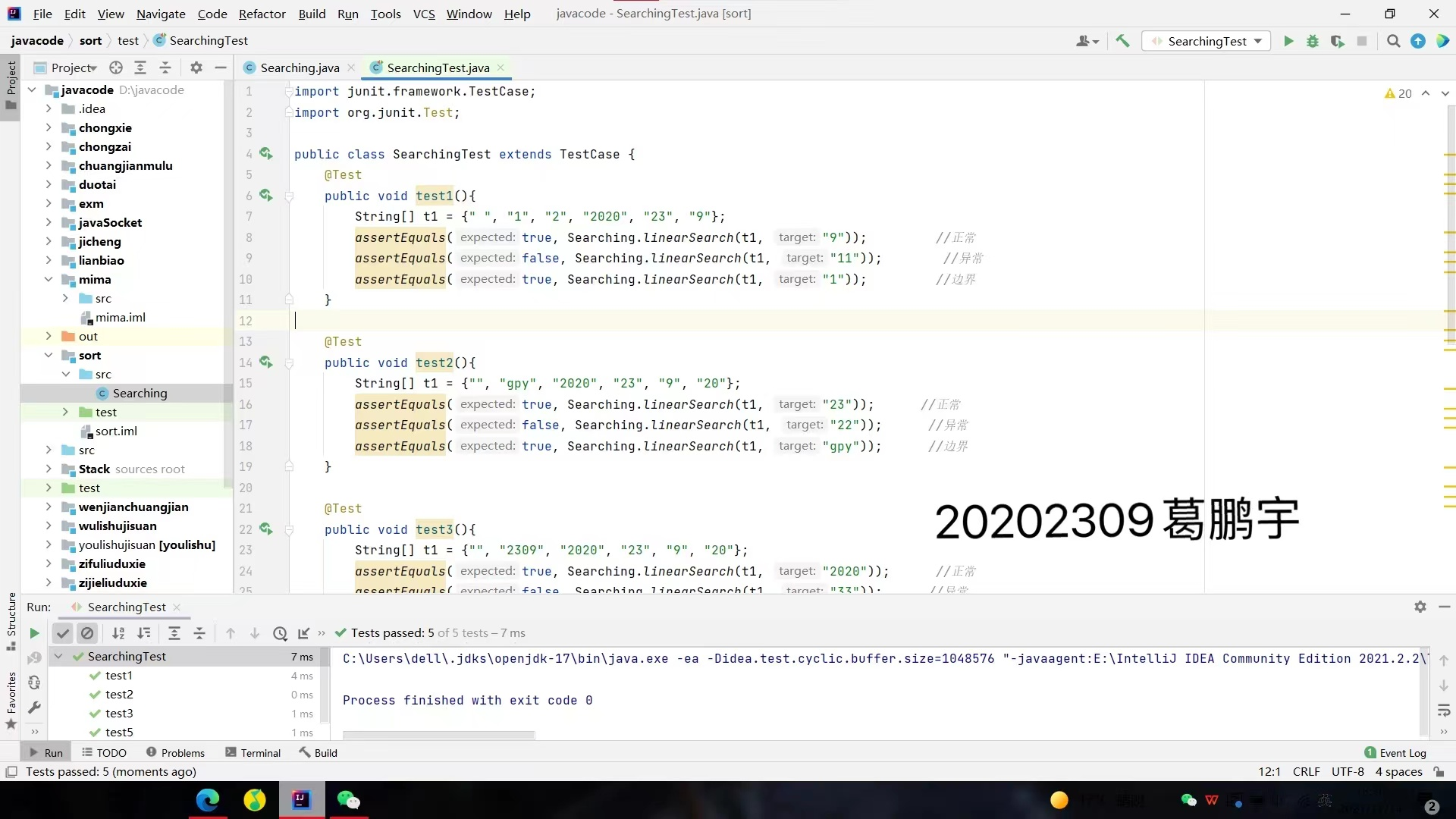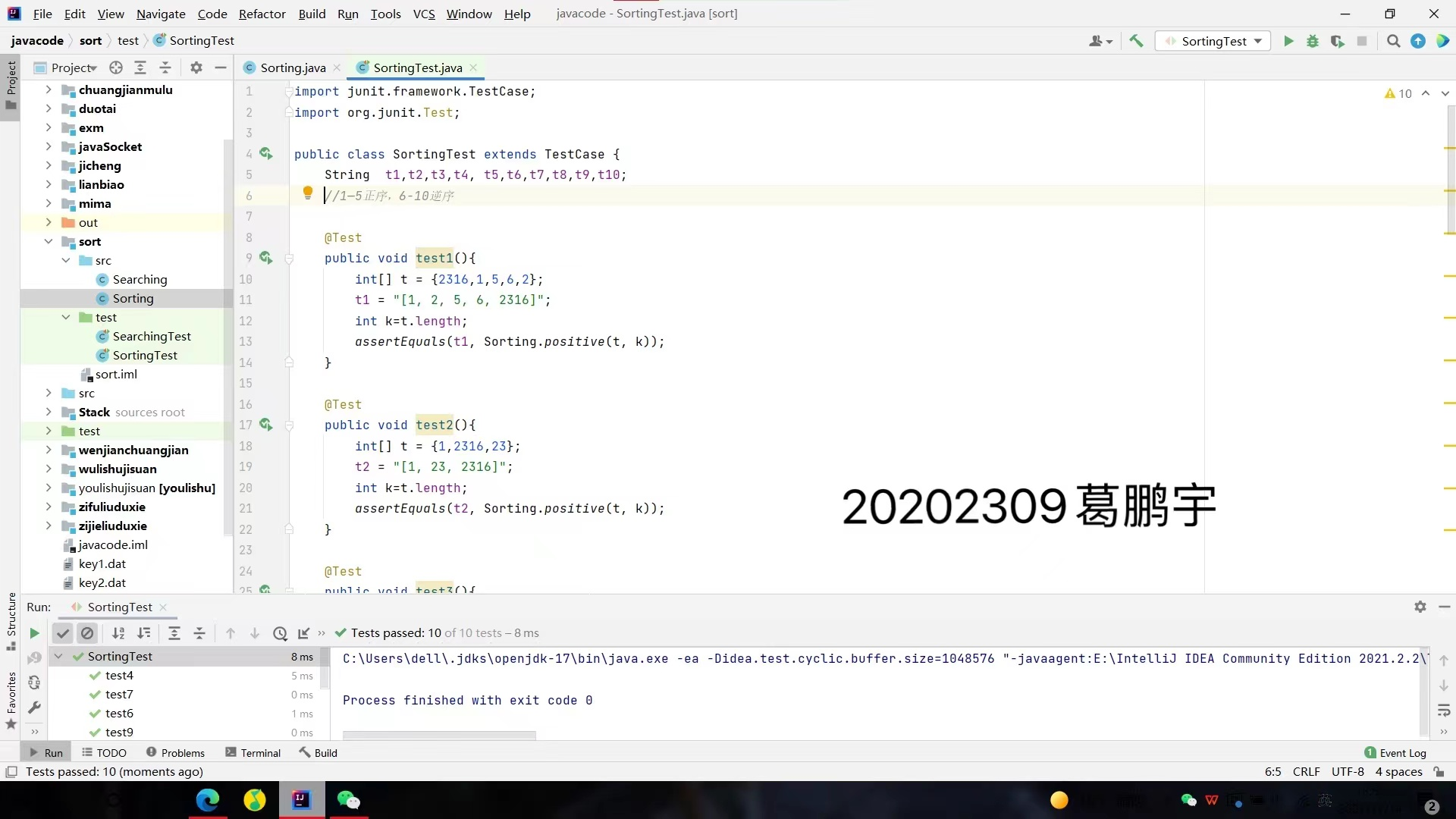2、 重构你的代码

把Sorting.java Searching.java放入 cn.edu.besti.cs2023.（姓名首字母+四位学号） 包中（例如：cn.edu.besti.cs1823.G2301）

把测试代码放test包中

重新编译，运行代码，提交编译，运行的截图（IDEA，命令行两种）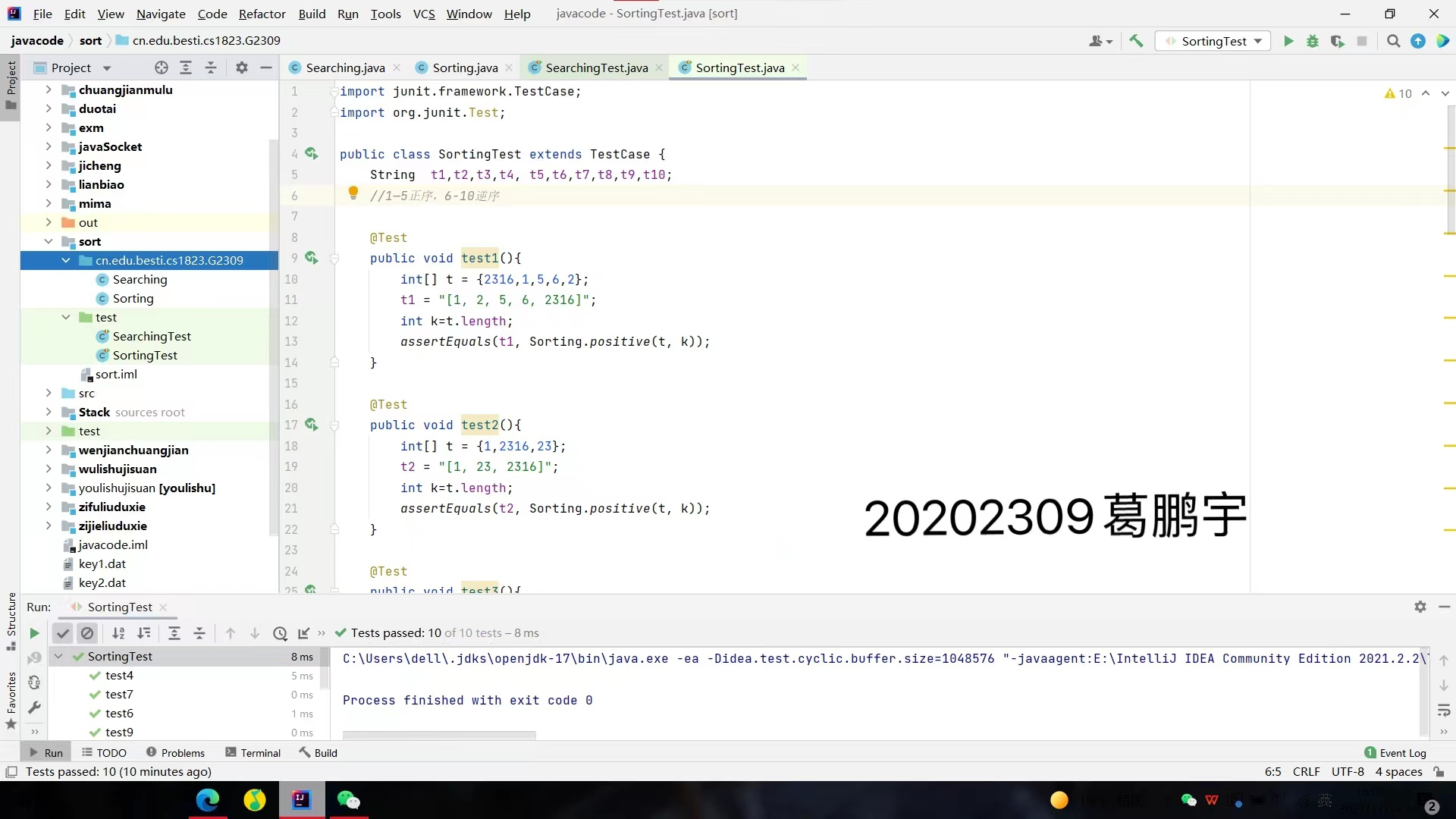idea中实现较为方便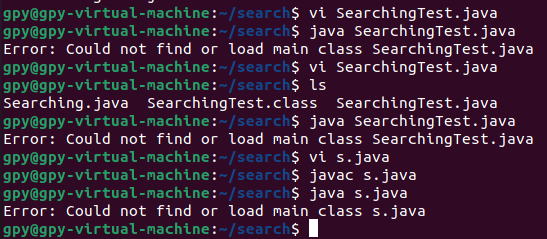尝试最简单的程序也运行不了，懵了。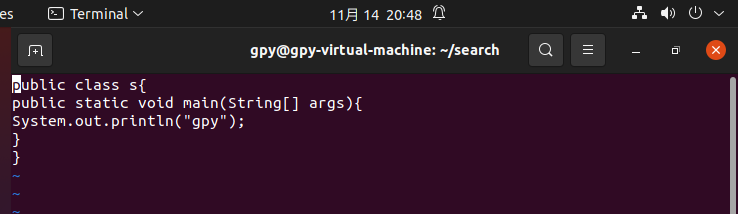3、参考http://www.cnblogs.com/maybe2030/p/4715035.html ，学习各种查找算法并在Searching中补充查找算法并测试

import java.util.Arrays;
public class Search1{
//二分
public static <T> int Search11(int a[], int value, int low, int high) {
int mid = low + (high - low) / 2;
if(a[mid] == value)
return mid;
else if(a[mid] > value)
return Search11(a, value, low, mid - 1);
else if(a[mid] < value)
return Search11(a, value, mid + 1, high);
else return -1;
}
//插值
public static <T> int Search22(int a[], int value, int low, int high)
{
int mid = low+(value - a[low]) / (a[high] - a[low]) * (high - low);
if(a[mid] == value)
return mid;
else if(a[mid] > value)
return Search22(a, value, low, mid-1);
else if(a[mid] < value)
return Search22(a, value, mid+1, high);
else return 0;
}
//斐波那契
public static void Fibonacci(int F[]){
int maxSize = 20;
F = 0;
F = 1;
for (int i = 2; i < maxSize; i++){
F[i] = F[i - 1] + F[i - 2];
}
}
public static <T> int fibonacciSearching(int a[], int target, int size){
int low = 0;
int high = size - 1;
int maxSize = 20;
int F[] = new int[maxSize];
Fibonacci(F);
int k = 0;
while (size > F[k] - 1){
k++;
}

int temp[]= Arrays.copyOf(a, F[k]);

for(int i = size; i < F[k] - 1; ++i){
temp[i] = a[size - 1];
}

while(low <= high) {
int mid = low + F[k - 1] - 1;
if (target < temp[mid]) {
high = mid - 1;
k -= 1;
} else if (target > temp[mid]) {
low = mid + 1;
k -= 2;
} else {
if (mid < size)
return mid;
else
return size - 1;
}
}
return -1;
}
//分块
public static int Search44(int[] index,int[]t,int key,int m){
int i = search(index,key);
if(i >= 0){
int j = m*i;
int site = (i + 1) * m;
for( ; j < site; j++){
if(t[j] == key)
return j;
}
}
return -1;
}
public static int search(int[]index,int keytype){
int i;
for(i = 0; ; i++){
if(index[i] >= keytype){
break;
}
}
return i;
}
//哈希
public static <T> int Search55(int[] a, int key){
int m = key % 11;
while(a[m] != key && m < a.length - 1){
m++;
}
if(a[m] != key){
return -1;
}
else{
return m;
}
}
}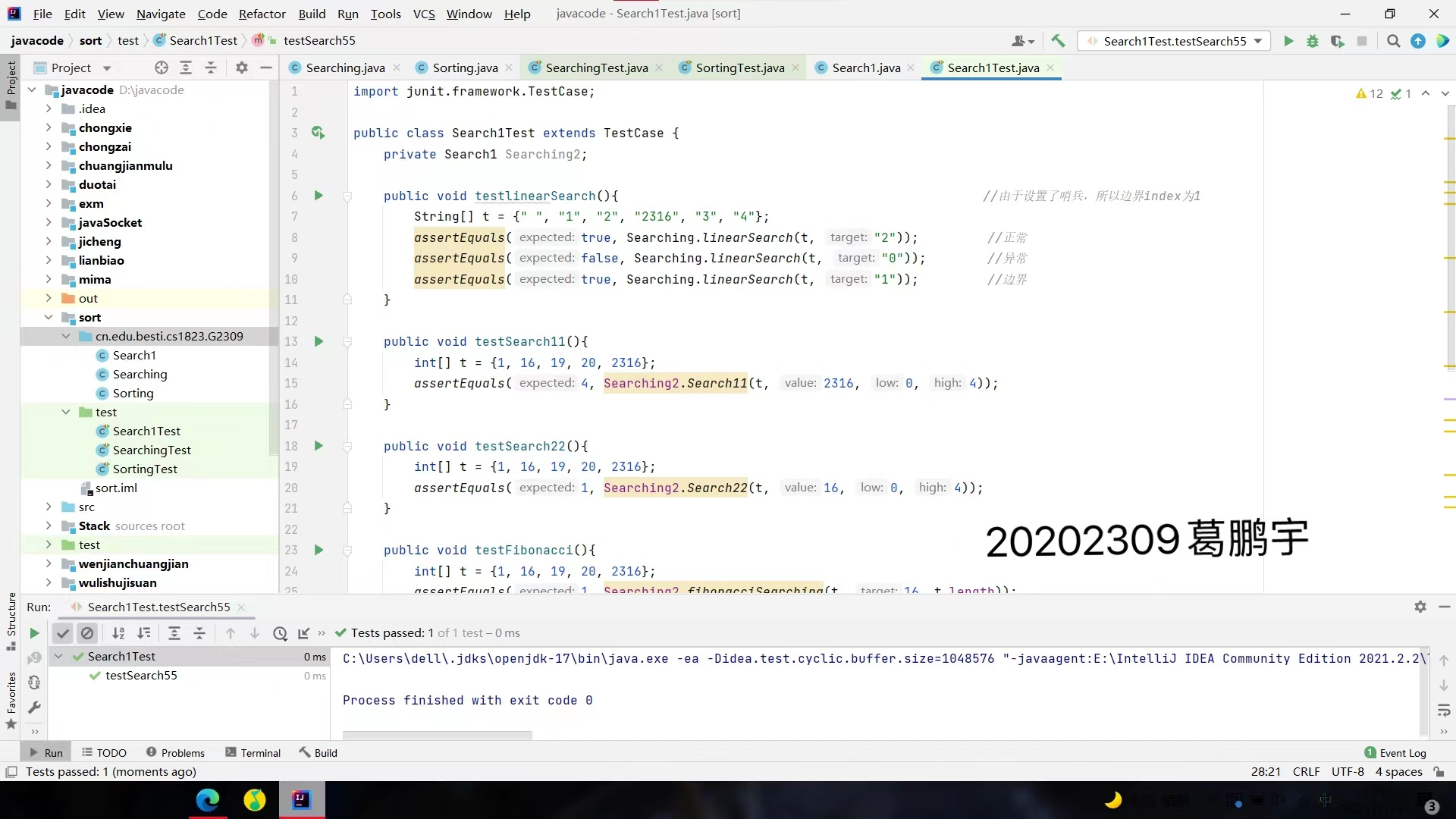4、实现排序方法等(至少3个)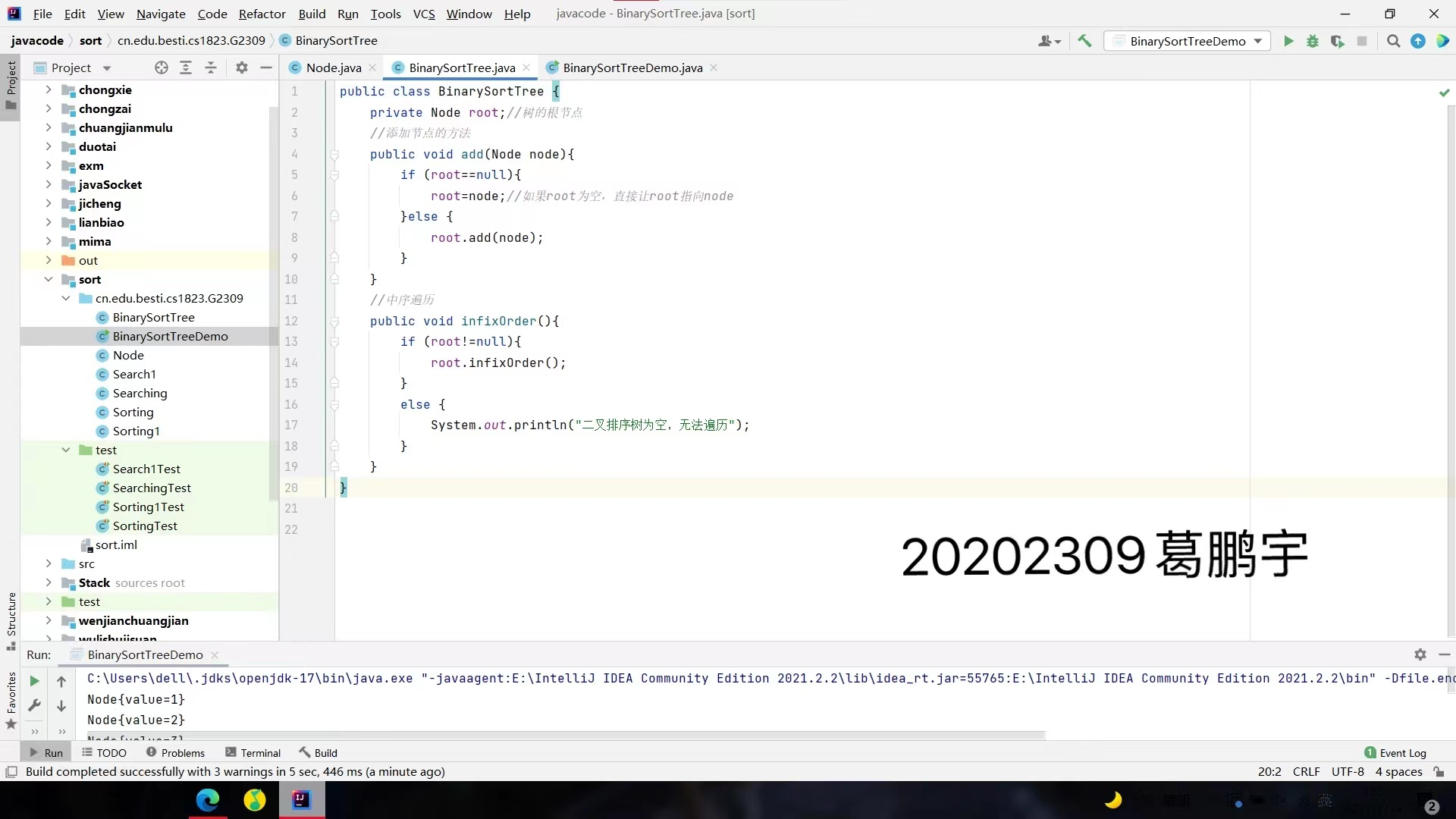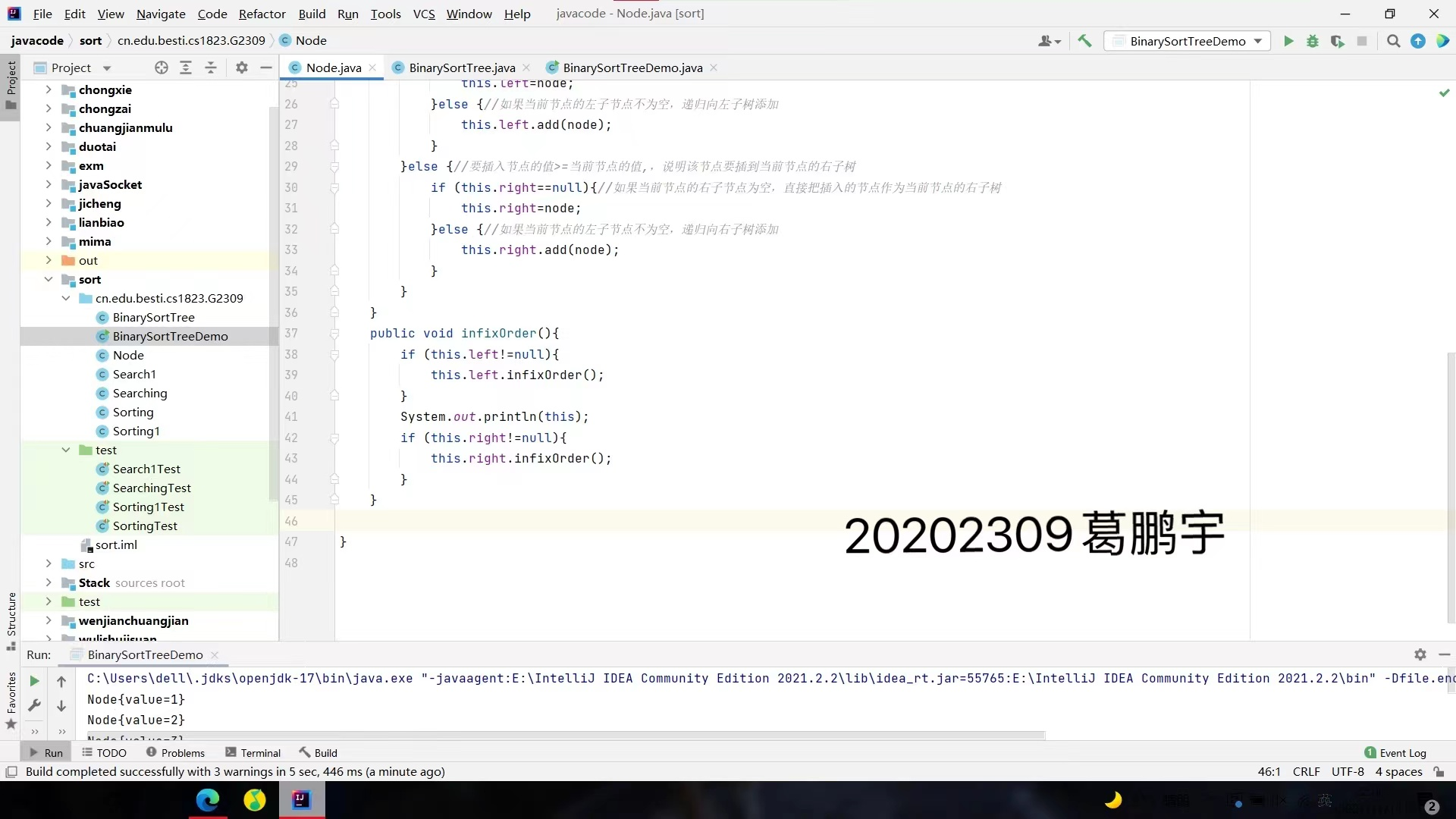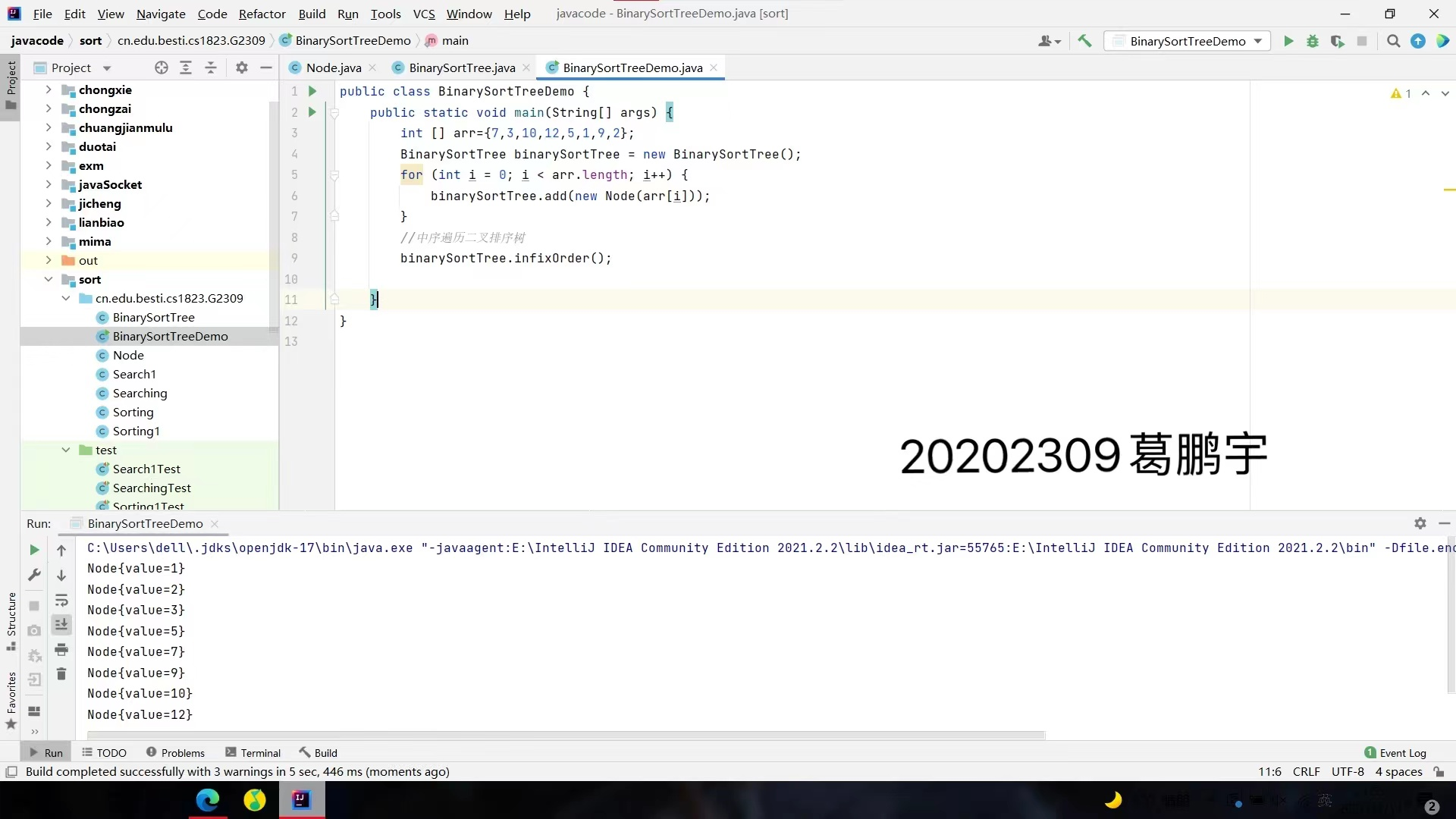希尔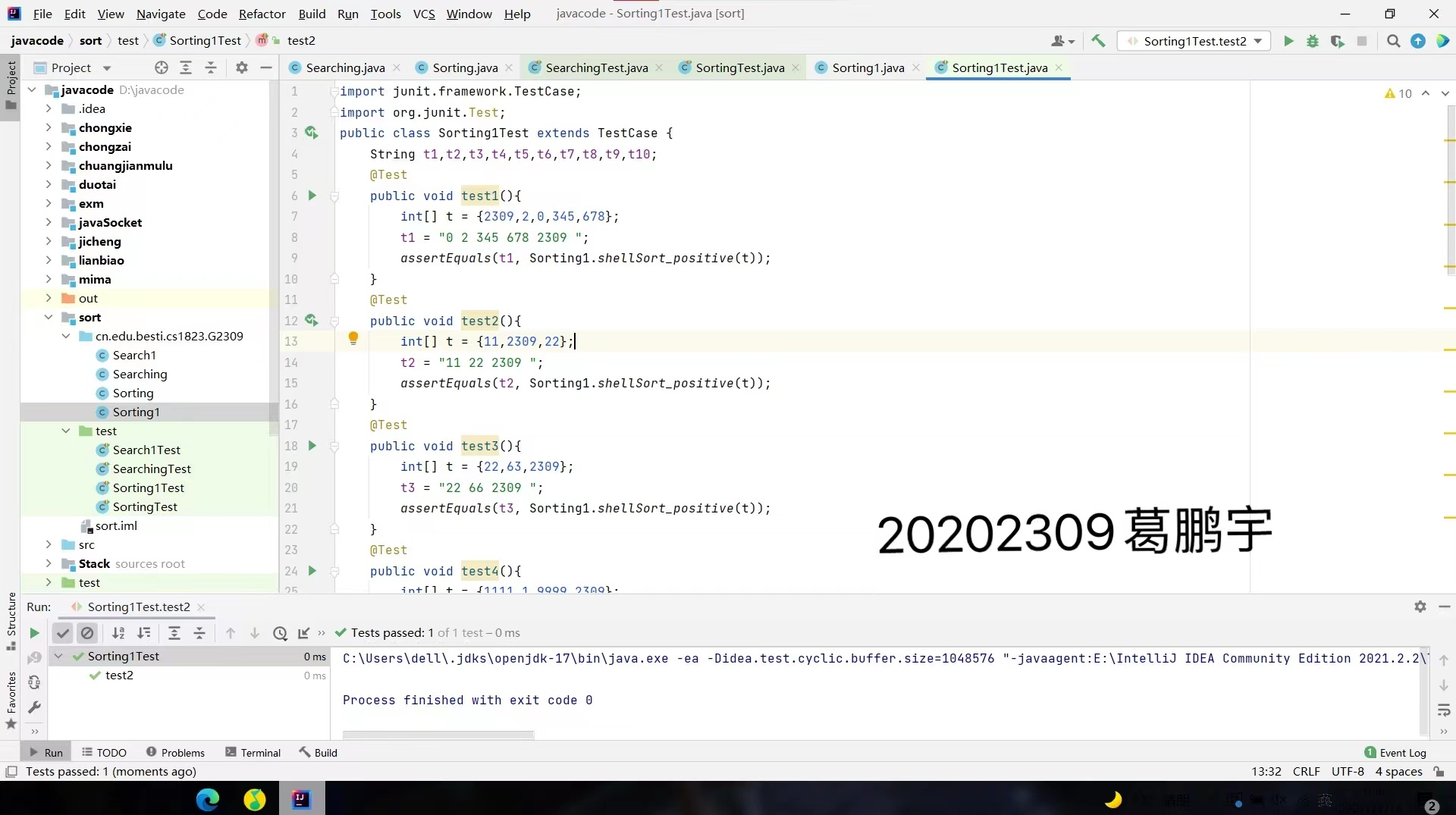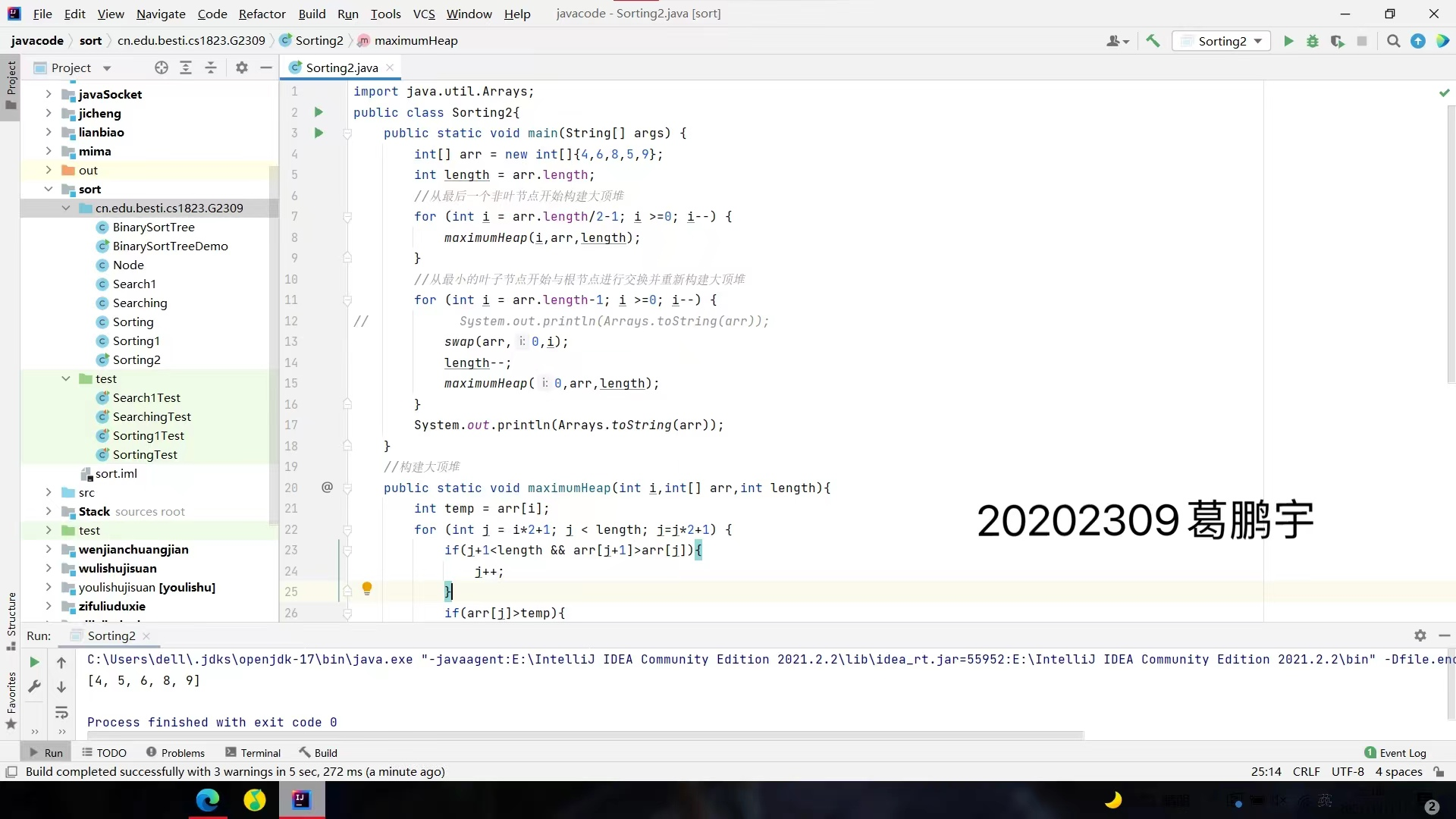## 3. 实验过程中遇到的问题和解决过程
- 问题1：linux系统Java程序无法识别main函数运行。

- 问题1解决方案：看了半天也没看出原因。暂未解决。

- 问题2：第四个实验题目二叉树查找不熟悉

- 问题2解决方案：通过网上查找别人的讲解暂时理解。

## 其他（感悟、思考等）

## 参考资料

-  [《Java程序设计与数据结构教程（第4版）》]

posted @ 2021-11-14 22:41  20202309葛鹏宇  阅读(7)  评论(0编辑  收藏  举报